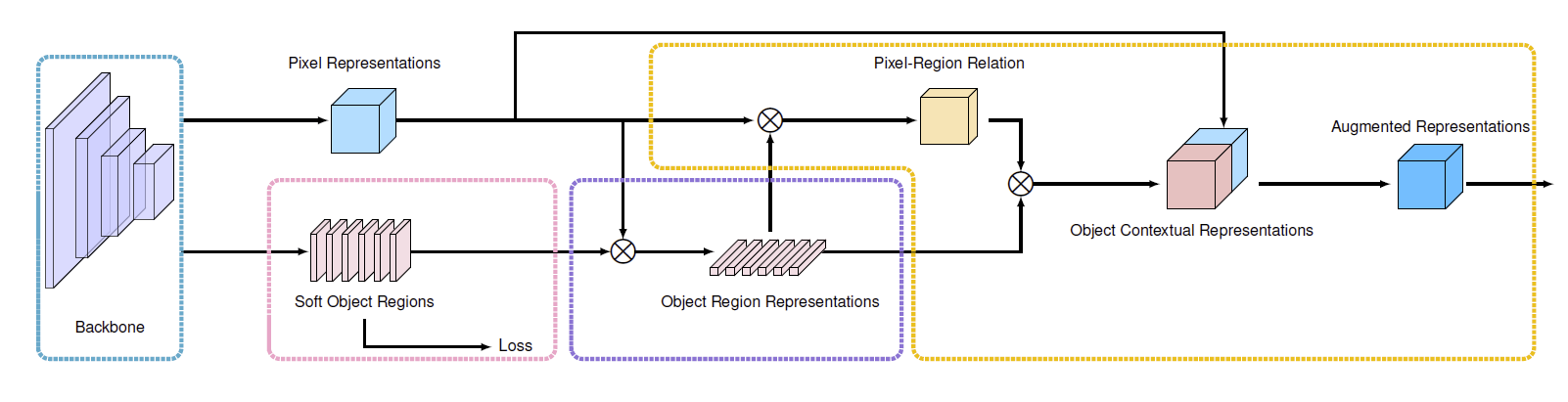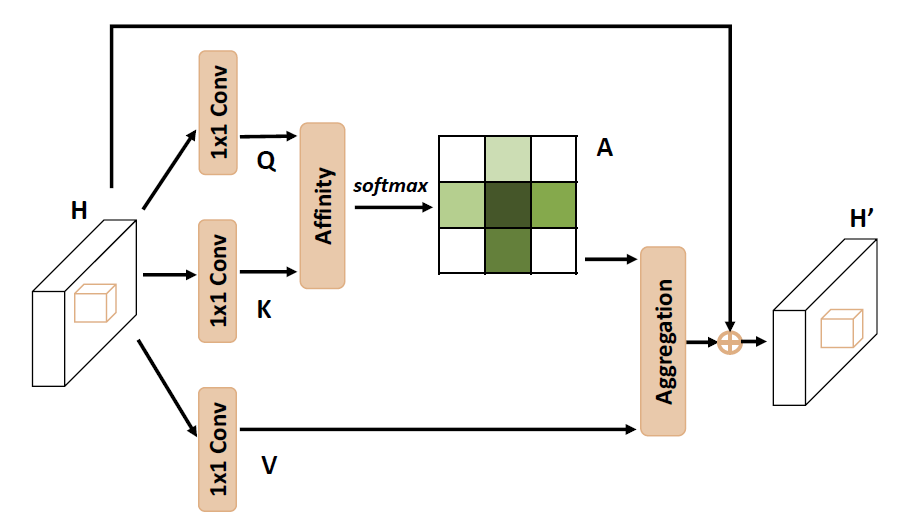Jarvis' Blog 总有美丽的风景让人流连

# 多尺度上下文信息 (Multi-Scale Context)

2022-02-14
Jarvis
Post

## 1. 概念

• CNN 中, 通过多层卷积和下采样可以扩大高层卷积核的感受野
• RNN 中, 通过多次重复循环单元实现远距离信息的融合

## 2. Non-Local Operation

Non-Local 操作的一般形式是 Non-Local Network1 提出的:

$\mathcal{y}_i = \frac1{\mathcal{C}(\mathbf{x})}\sum_{\forall j}f(\mathbf{x}_i, \mathbf{x}_j)g(\mathbf{x}_j),$

#### 2.1 二元函数 $f(\cdot, \cdot)$

• 高斯函数:

$f(\mathbf{x}_i, \mathbf{x_j}) = e^{\mathbf{x}_i^T\mathbf{x}_j}.$
• 嵌入的高斯函数:

$f(\mathbf{x}_i, \mathbf{x}_j) = e^{\theta(\mathbf{x}_i)^T\phi(\mathbf{x}_j)}.$

这里的 $\theta(\mathbf{x}_i)$ 和 $\phi(\mathbf{x}_j)$ 可以是线性映射, 也可以是其他的复杂函数.

• 点积:

$f(\mathbf{x}_i, \mathbf{x}_j) = \theta(\mathbf{x}_i)^T\phi(\mathbf{x}_j).$
• 拼接:

$f(\mathbf{x}_i, \mathbf{x}_j) = \text{ReLU}(\mathbf{w}^T_f[\theta(\mathbf{x}_i), \phi(\mathbf{x}_j)]).$

## 3. ASPP, PSPNet

• ASPP2: 通过一系列并行的不同膨胀率的膨胀卷积实现. 详见 DeepLab 系列.
• PSPNet3: 通过 Pyramid Pooling Module 在不同尺度的特征图上做常规卷积来捕获多尺度信息, 但是损失了特征图的精度. 详见 PSPNet 系列.

## 4. Self-Attention

Self-Attention4 通过计算目标 pixel 和其他所有 pixels 之间的关系作为 attention map (权重), 再通过特征的加权和来吸收上下文的信息.

$\label{eq:ocr} \mathbf{y}_i = \rho(\sum_{s\in\mathcal{I}} w_{is}\delta(\mathbf{x}_s))$

## 5. Object-Context Representation (OCR)

OCR5 的 motivation 是表示图像中不同的 object, 然后每个 pixel 从 objects 的表示中吸收信息.#### 5.1 Object 区域的表示

$\mathbf{f}_k = \sum_{i\in\mathcal{I}} \tilde{m}_{ki}\mathbf{x}_i,$

#### 5.2 OCR

$w_{ik} = \frac{e^{\kappa(\mathbf{x}_i, \mathbf{f}_k)}}{\sum_{j=1}^K e^{\kappa(\mathbf{x}_i, \mathbf{f}_j)}}$

#### 5.3 增广的特征表示

$\mathbf{z}_i = g(\text{cat}(\mathbf{x}_i, \mathbf{y}_i)),$

## 6. Criss-Cross Attention (CCA)CCA 的复杂度为 $\mathcal{O}(N\sqrt{N})$.

## 参考文献

1. Non-local neural networks>
Xiaolong Wang, Ross Girshick, Abhinav Gupta, Kaiming He
[html] In CVPR 2018

2. DeepLab: Semantic Image Segmentation with Deep Convolutional Nets, Atrous Convolution, and Fully Connected CRFs
Liang-Chieh Chen, George Papandreou, Iasonas Kokkinos, Kevin Murphy, and Alan L Yuille.
[html]. In TPAMI 2017.

3. Pyramid Scene Parsing Network
Hengshuang Zhao, Jianping Shi, Xiaojuan Qi, Xiaogang Wang, Jiaya Jia
[html] In CVPR 2017

4. Attention is all you need
Ashish Vaswani, Noam Shazeer, Niki Parmar, Jakob Uszkoreit, Llion Jones, Aidan N. Gomez, Lukasz Kaiser, Illia Polosukhin
[html] In NeurIPS 2017

5. Segmentation Transformer: Object-Contextual Representations for Semantic Segmentation
Yuhui Yuan, Xiaokang Chen, Xilin Chen, and Jingdong Wang
[html] In ECCV 2020

6. CCNet: Criss-Cross Attention for Semantic Segmentation>
Zilong Huang, Xinggang Wang, Yunchao Wei, Lichao Huang, Humphrey Shi, Wenyu Liu, Thomas S. Huang
[html] In ICCV 2019 and TPAMI 2020

Content